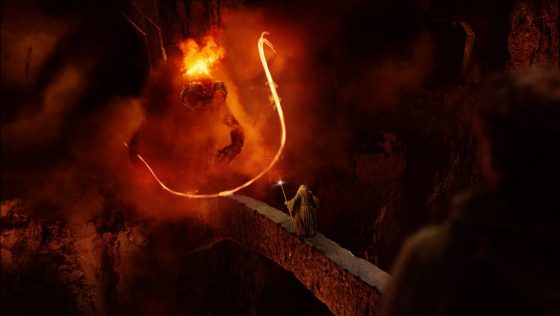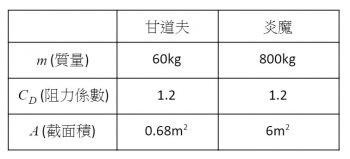# 《這不科學！——魔戒中的數學思辯》——2019數感盃 高中職組專題報導類佳作

2019/05/24 | | 標籤：

• 作者：王治鈞、呂建霆、洪林竹／國立科學工業區實驗高級中學。## 序章

1. 伽利略告訴我們，在同個高度下，不同物體掉到地上的時間皆應相同
2. 炎魔比甘道夫更早開始下墜
3. 更精確地說，炎魔早了32秒

## 加入「空氣阻力」的條件

1. 寫出甘道夫和炎魔墜落時所必須遵守的運動方程式
2. 根據電影本身和其他資料估計一些參數（像是質量、空氣密度等）
3. 解微分方程
4. 把之前估計的參數代回式子中解完方程
5. 回答我們提出來的問題——甘道夫和炎魔到底會不會同時墜地？

$$F = \frac{1}{2}ρC_DAv^2$$

$$ma$$$$=m\frac{dv}{dt}$$$$=F-mg$$$$=\frac{1}{2}ρC_DAv^2-mg$$$$γ\equiv \frac{1}{2m}ρC_DA$$

，所以對於甘道夫和炎魔來說，他們的 γ 分別是 0.0088 和 0.0058。

$$\frac{dv}{dt}$$$$=γv^2-g, \int \frac{dv}{γv^2-g}$$$$=\int dt$$

$$\frac{1}{2\sqrt{γg}}(ln(\sqrt{\frac{g}{γ}}-v)-ln(v+\sqrt{\frac{g}{γ}}))$$$$=t+t_0$$

$$v=-\sqrt{\frac{g}{γ}} \tan{h}\tan{h}[\sqrt{gγ}(t+t_0)]$$

$$y=\int_{0}^{t}vd{t}’$$$$=-γ^{-1}\ln \ln\cos{h}\cos{h}\sqrt{gγt}$$

t0是積分常數，如果把t=0設定成開始墜落的時間，那麼 t應該是0，這樣一開始的速率才會是0 （因為 tanh0=0）。底下兩個式子中的第一個告訴我們當一個法師（或是某種遠古怪獸）墜入深淵時，速度和時間的關係；而第二個則是掉落距離和時間的關係。我們需要知道的只有 γ，而這和掉落物本身的性質有關。

，甘道夫才開始往下掉。

## 關於炎魔的鞭子

$$y=\int_{0}^{t}vd{t}’=-γ^{-1}\ln \ln\cos{h}\cos{h}\sqrt{gγt}$$

$$0.0058^{-1}\ln\ln\cos{h}\cos{h}(\sqrt{g\cdot 0.0058\cdot 14})$$$$\approx 460(m)$$

，才有機會在墜落後 14 秒將鞭子伸上來勾住甘道夫。

## 結論

### 參考資料

1. Tolkien Gateway
2. Koo, M. H., & Al-Obaidi, A. S. M. (2013). Calculation of aerodynamic drag of human being in various positions. Department of Mechanical Engineering, Taylor’s University, Malaysia.
3. McIlveen, J. F. R. (2002). The everyday effects of wind drag on people. Weather57(11), 410-413.

## 關於作者